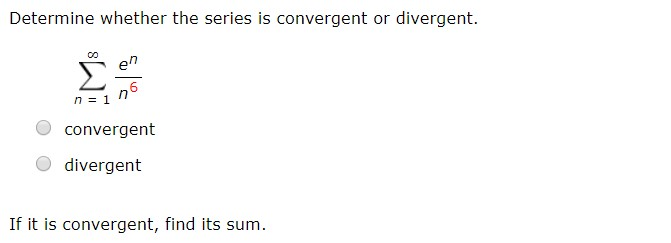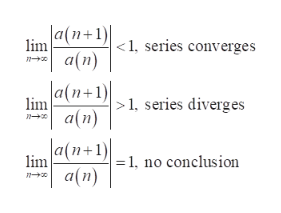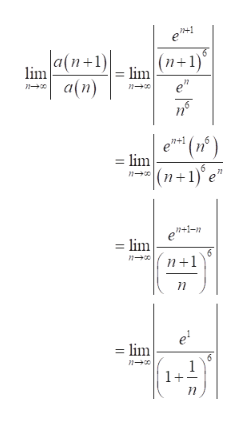# Determine whether the series is convergent or divergent.enn1convergentdivergentIf it is convergent, find its sum.

Question
4 viewshelp_outlineImage TranscriptioncloseDetermine whether the series is convergent or divergent. en n1 convergent divergent If it is convergent, find its sum. fullscreen
check_circle

Step 1

According to ratio test ,the sum of series, a(n) from 1 to infinity converges or diverges according to the following condition.help_outlineImage TranscriptioncloseJa(n+1) lim a(n) <1, series converges a(n+1) a(n) 1, series diverges lim a(n+1) = 1, no conclusion lim fullscreen
Step 2

Identify the function,

Step 3

Substitute the function a(n) in t...help_outlineImage Transcriptionclose1 a(n+1)| (n+1) lim e" lim a(n) (n) |(n+1)'e° +1 = lim n lim lim 6 1+ fullscreen

### Want to see the full answer?

See Solution

#### Want to see this answer and more?

Solutions are written by subject experts who are available 24/7. Questions are typically answered within 1 hour.*

See Solution
*Response times may vary by subject and question.
Tagged in